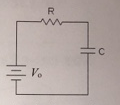# Problem: For RC circuit shown here, Kirchhoff's voltage loop rule would result in V0 – IR – Q/C = 0. If the capacitor initially has a charge Q0 on it at time 0 (it is partially pre-charged), the charge on it at later times is given by Q = V0C(1 – e-t/RC) + Q0e-t/Rc. This expression is a combination of the capacitor both charging and discharging simultaneously. i) Find the current in the circuit by differentiating the above expression for charge. ii) Show that with the expressions for charge as given in the statement of the problem, and the current that you found in part i), that Kirchhoffs loop equation [V0 – IR – Q/C = 0] is satisfied.

###### FREE Expert Solution

i) We are told to differentiate the expression for Q.

Power rule of derivation:

$\overline{)\frac{\mathbf{d}}{\mathbf{d}\mathbf{t}}\mathbf{\left(}{\mathbf{e}}^{\mathbf{-}\mathbf{n}\mathbf{t}}\mathbf{\right)}{\mathbf{=}}{\mathbf{-}}{\mathbf{n}}{{\mathbf{e}}}^{\mathbf{-}\mathbf{n}\mathbf{t}}}$

The given expression is:

$\begin{array}{rcl}Q& =& {\mathbit{V}}_{\mathbf{0}}\mathbit{C}\mathbf{\left(}\mathbf{1}\mathbf{-}{\mathbit{e}}^{\mathbf{-}\mathbit{t}\mathbf{/}\mathbf{RC}}\mathbf{\right)}\mathbf{+}{\mathbit{Q}}_{\mathbf{0}}{\mathbit{e}}^{\mathbf{-}\mathbit{t}\mathbf{/}\mathbf{RC}}\\ & =& {\mathbit{V}}_{\mathbf{0}}\mathbit{C}\mathbf{-}{\mathbit{V}}_{\mathbf{0}}\mathbit{C}{\mathbit{e}}^{\mathbf{-}\mathbit{t}\mathbf{/}\mathbf{RC}}\mathbf{+}{\mathbit{Q}}_{\mathbf{0}}{\mathbit{e}}^{\mathbf{-}\mathbit{t}\mathbf{/}\mathbf{RC}}\end{array}$

93% (434 ratings)###### Problem Details

For RC circuit shown here, Kirchhoff's voltage loop rule would result in V0 – IR – Q/C = 0. If the capacitor initially has a charge Q0 on it at time 0 (it is partially pre-charged), the charge on it at later times is given by Q = V0C(1 – e-t/RC) + Q0e-t/Rc. This expression is a combination of the capacitor both charging and discharging simultaneously.i) Find the current in the circuit by differentiating the above expression for charge.

ii) Show that with the expressions for charge as given in the statement of the problem, and the current that you found in part i), that Kirchhoffs loop equation [V0 – IR – Q/C = 0] is satisfied.